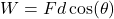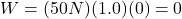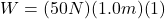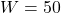## 10. How much total work do you do when you lift a 50 kg microwave 1.0 m off the ground and then push it 1.0 m across a counter with a

Question

10. How much total work do you do when you lift a 50 kg microwave 1.0 m off the ground and then push it 1.0 m
across a counter with a force of 50 N?

in progress 0
5 months 2021-09-05T10:28:16+00:00 1 Answers 6 views 0

1. Work formula:F = 50N, d = 1.0 m

When you lift something straight up, the angle of the force is 90º

cos(90º) is 0, so there’s no work done when you lift the microwave off the groundF = 50N, d = 1.0 m

When you push the microwave, the angle is 0º and cos(0º) is 1. So there is work done here:total work = 50 joules